Function Repository Resource:

# CoinTossSimulator

Dynamically simulate tossing a coin

Contributed by: Wolfram|Alpha Math Team
 ResourceFunction["CoinTossSimulator"][] creates an interface for dynamically simulating coin tosses. ResourceFunction["CoinTossSimulator"][w] uses probability w of getting "heads".

## Details and Options

ResourceFunction["CoinTossSimulator"] returns a DynamicModule expression.
The returned interface includes the buttons Flip, Report and Ideal results. These simulate a coin toss and use CellPrint to display a report of the current results and a plot comparing the results to ideal results, respectively.

## Examples

### Basic Examples (1)

Simulate tossing a fair coin:

 In:=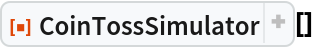Out=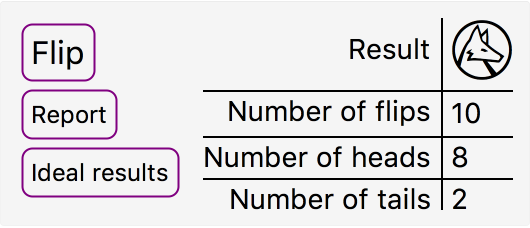### Scope (2)

Simulate an unfair coin:

 In:=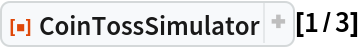Out=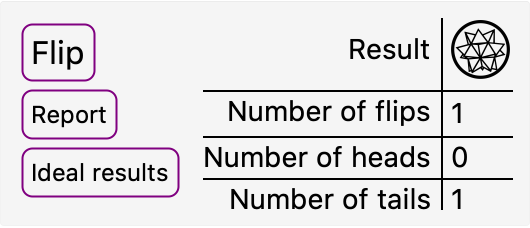Clicking the "Report" button returns a summary of the simulation statistics:

 In:=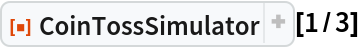Out=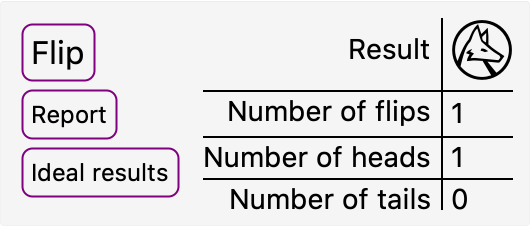Out=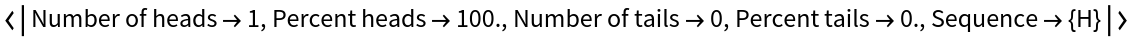### Properties and Relations (1)

The information returned by the "Ideal results" button is also available via the resource function IdealCoinTossStatistics:

 In:=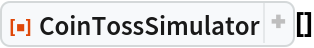Out=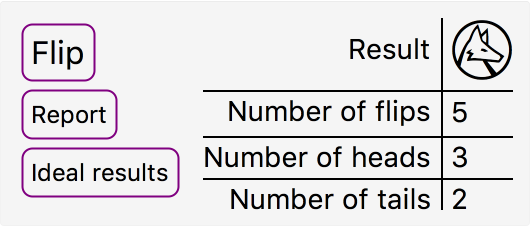Out=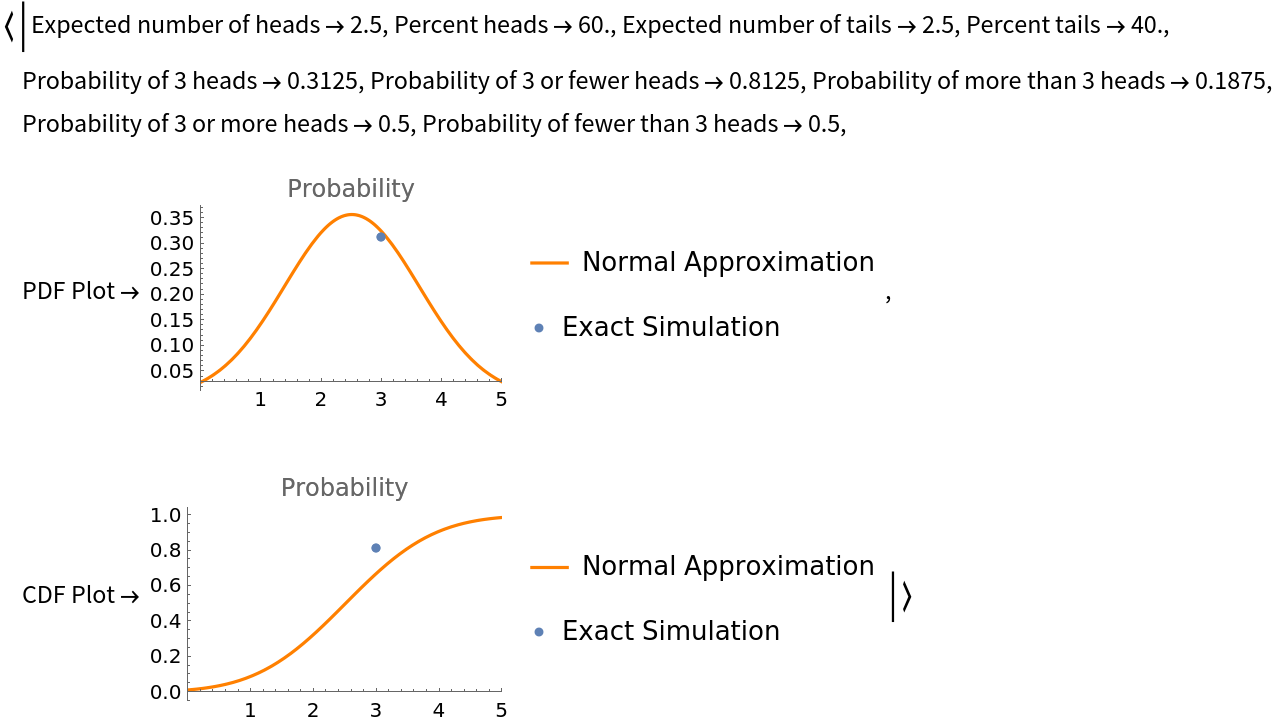In:=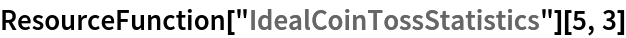Out=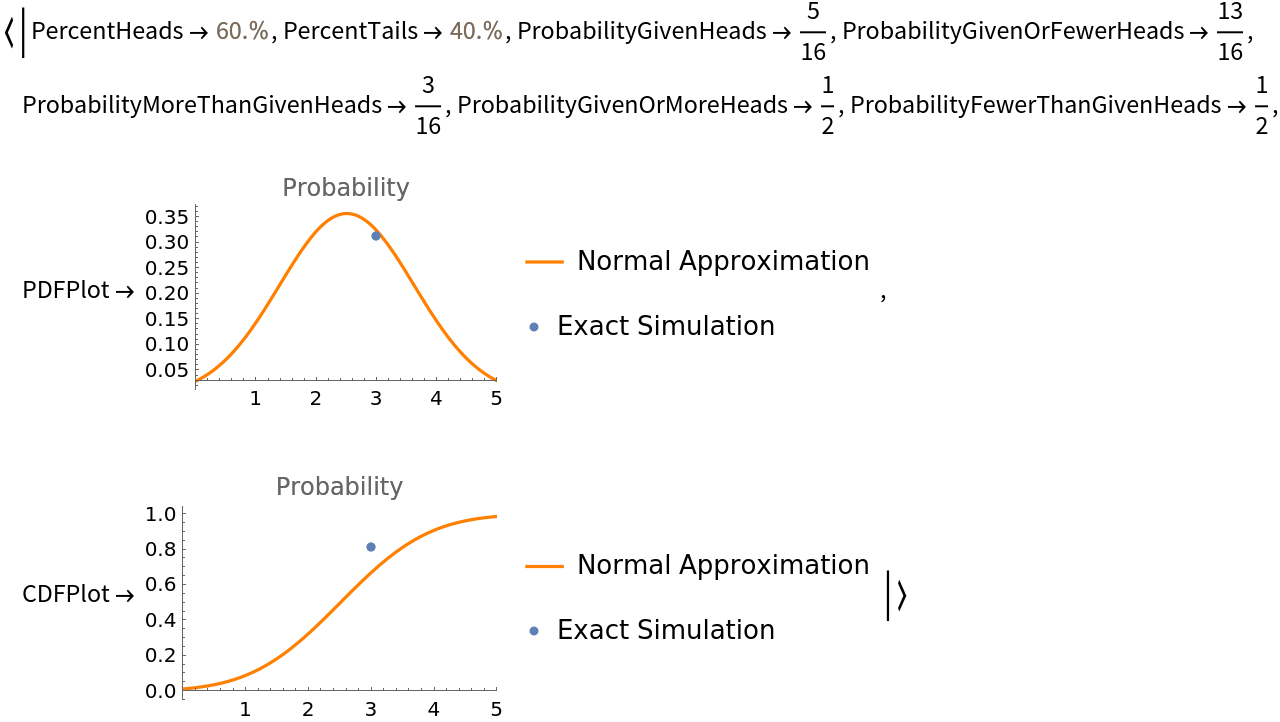## Publisher

Wolfram|Alpha Math Team

## Version History

• 2.0.0 – 23 March 2023
• 1.0.0 – 18 September 2020

## Author Notes

To view the full source code for CoinTossSimulator, run the following code:

 In:=